Related Articles

# Julia – DataFrames

• Last Updated : 28 Jul, 2020

Data Frames in Julia is an alternative for Pandas Package in Python. Data Frames represent the data in a tabular structure. We can manipulate the data using these data frames. Various operations can be done on the Data frames for altering the data and making row-column transformations.

Data Frames are mainly used and created for accessing the data in a row-column manner.

Similarly to the python installation of packages, Julia also includes the importing methods.

#### Installation of required packages:

Julia can be programmed using Jupyter notebooks or Atom software. To install data frame package in Julia, please use the following commands:

```using Pkg

Momentarily, DataFrame in Julia is covered in the program by importing them by the keyword argument “using”.  The data is represented in a row-column manner and manipulated using the operations that we come across in later read.  To import DataFrames package in your code, use the following command:

```using DataFrames
```

#### Creation of DataFrames

Data frames in Julia are created with the use of pre-defined DataFrame() function. It takes values and column names as argument and creates a data frame.

Example:

## Julia

 `# Creation of a Data Frame``dataframe ``=` `DataFrame(I ``=` `[``17.20``, ``22.30``], II ``=` `[``49``, missing], III ``=` `[``"Hello"``, ``"Nicy"``])`

Output: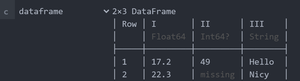DataFrame of order 2×3

As explained above, we got to know all about creating a data frame in Julia. Let us understand the structure of the data frame created above by understanding the row-column structured data.

The columns here are  I, II, and III they act as user-defined keywords for representing the columns.

1. The Column “I” comprises of float values.
2. The Column “II” includes an integer value and a missing value.
3. The Column “III” holds String characters.

#### Accessing columns of a Data frame

In Julia, columns can be accessed through “data.column” here ‘data’ is our variable element in which we have created our DataFrame and ‘column’ is the user-defined keyword for our DataFrame column.

First, let us create a data frame to perform further operations on it

## Julia

 `# Creating a Data frame``dataframe2 ``=` `DataFrame(I ``=` `1``:``5``, II ``=` `[``"True"``, ``"False"``, ``"False"``, ``"True"``,``"False"``],``                       ``C ``=` `[``"Approved"``, ``"Dissapproved"``, ``"Approved"``,``                            ``"Approved"``,``"Dissapproved"``])`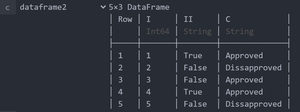dataframe of order 5×3

Let us now look at the examples of accessing the column elements using some of the operations.

## Julia

 `# Accessing second column``op1 ``=` `dataframe2.II`

We have accessed the second column in the dataframe2 object created and stored the retrieved column in the ‘op1’ variable.

Output: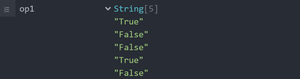2nd column

## Julia

 `# Accessing using double quotes``op2 ``=` `dataframe2.``"II"`

As we can compare with the above code in which we accessed column two. Similarly, we can also access any column by including them in double-quotes.

Output: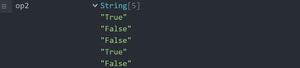2nd column

## Julia

 `# Accessing first column``op3 ``=` `dataframe2[!, :I]`

The syntax denotes the accessing of the first column

Output: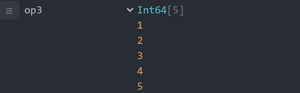column 1

## Julia

 `# Getting names of columns`` ` `# Using names() function``op4 ``=` `names(dataframe2)`` ` `# Using propertynames() function``op5 ``=` `propertynames(dataframe2)`

The above code represents two basic functions in Julia ‘names’ and ‘propertynames’.

• In Julia, the function ‘names’ display the column names in the dataframe created by the user.
• The function ‘propertynames’ display the column names as symbols using  ‘ : ‘ .

Output: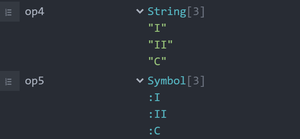names and propertynames

#### Adding elements to the DataFrame

Here, we are creating a data frame with no information instead declaring the datatype of the columns.

## Julia

 `# Creating an empty data frame``data ``=` `DataFrame(first ``=` `Int``[], sec ``=` `String[])`

Now that we have created a Data Frame and stored in a variable called ‘data’.

• The first column includes the data of integer datatype.
• The second column includes the data of String datatype.

Let us now push the data into the DataFrame columns by taking action with the ‘push()‘ function.

## Julia

 `# Adding first column``push!(data, (``17``, ``"Cat"``))`

Output: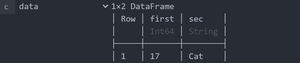data is pushed into dataframe

#### Renaming the columns of the DataFrame

In Julia, the renaming operation is done by a function rename!(). This function changes the name or the keyword of a column which is already been listed by the user.

## Julia

 `# Renaming columns of a data frame``ren ``=` `rename!(dataframe2, :I ``=``> :first)`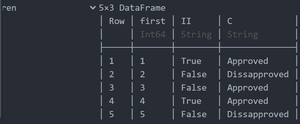The name of the I column is changed to first

#### Creating Subsets of a Data Frame

Subsets can be easily created by breaking the data frame into Head and Tail. The head of a Data frame can be displayed with the use of head() function and the tail can be displayed with the use of tail() function.

This function illustrates the head part of the DataFrame we created.

## Julia

 `# Displaying the head of a Data frame`` ` `# Using head() function``head1 ``=` `head(dataframe2)`

The head function returns us the head portion or the top part of our DataFrame.

Output: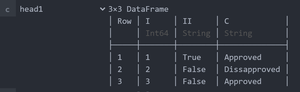Tail Function:

The tail function illustrates the tail part of the DataFrame created.

## Julia

 `# Displaying the tail of a data frame`` ` `# Using the tail() function``tail1 ``=` `tail(dataframe2)`

For the DataFrame created we have worked on the tail operation. The function displays bottom-most values in the data frame.

Output: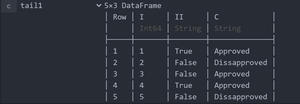The tail part of the dataframe

#### Deleting Rows and Columns of the DataFrame

The delete operation in Julia is performed using the delete!() function. This function takes data frame name and the row or column to be deleted as argument and performs the deletion.

## Julia

 `# Deleting a row of a data frame`` ` `# Using delete!() function``delete1 ``=` `delete!(dataframe2, ``4``:``5``)`

As declared in the above code we performed the delete operation on the ‘dataframe2’ and deleted the 4th row.

Output: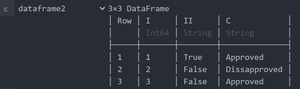DataFrame after deleting a row

Now that we have come across deleting a row using the function ‘delete!()‘.

Let us now eliminate a column element:

## Julia

 `# Deleting a column of a data frame`` ` `# Using delete!() function``del` `=` `select!(dataframe2, Not(:II))`

In the above code, we have accessed the columns of Dataframe2 excluding the ‘II’ column.

Output: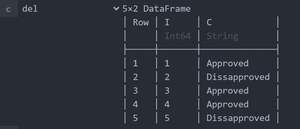Dataframe excluding 2nd column

My Personal Notes arrow_drop_up